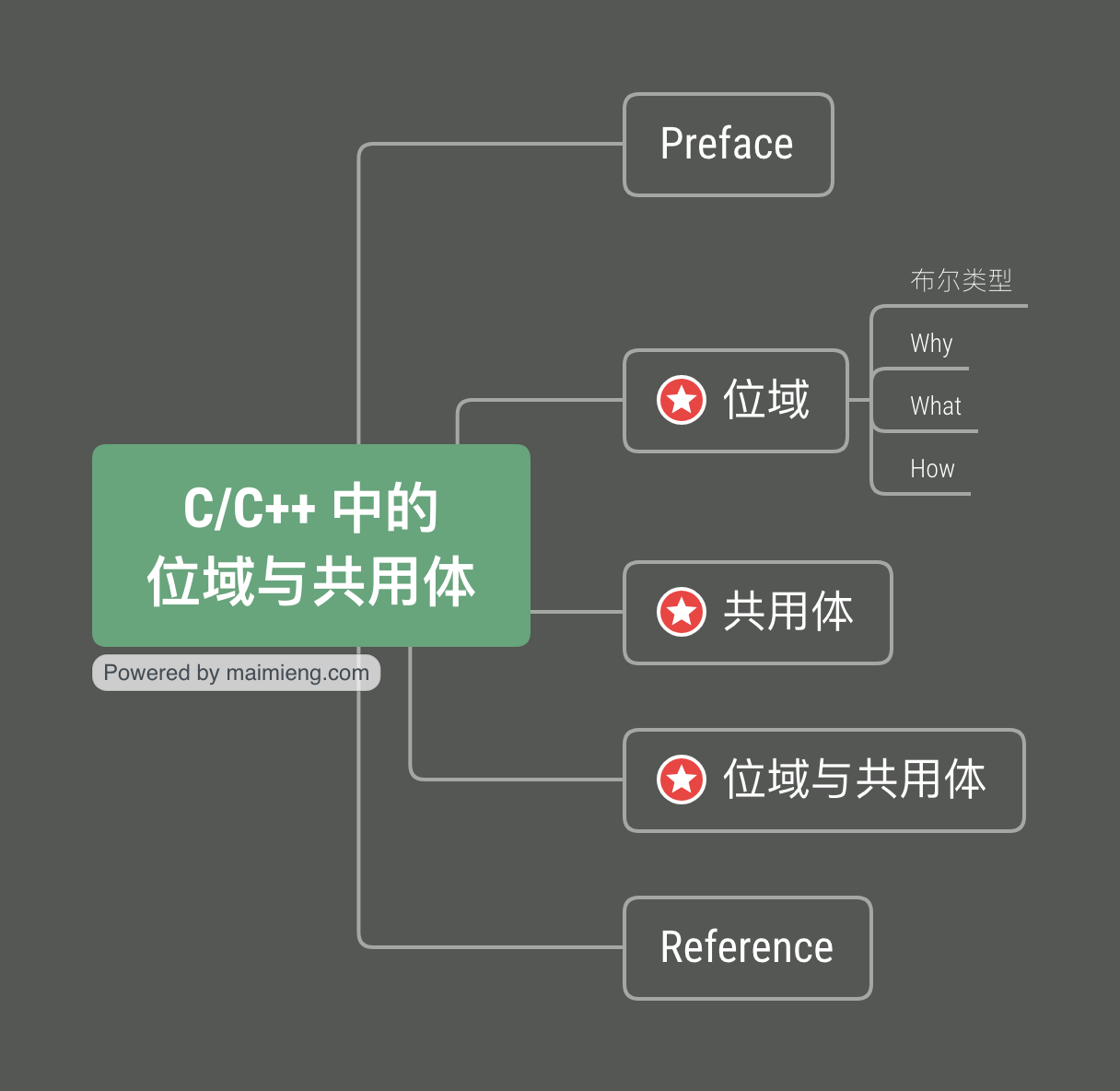## C/C++ 中的位域与共用体

2019.08.17 by kingcos
Date Notes Env
2019-08-17 首次提交 clang++、macOS 10.14.4## Preface

C/C++ 中有许多「奇技淫巧」来让开发者能够以效率更高的方式使用内存，这也是一些高级语言着力去避讳的一点，但这种能够直面内存细节的「踏实感」让我个人觉得非常安心。本文将简单涉及 C/C++ 中的位域（Bit Field）与共用体（Union）的概念。

## 位域

### 布尔类型

#include <iostream>

using namespace std;

int main()
{
bool foo = true;
bool bar = 0;

cout << foo << endl; // 1
cout << bar << endl; // 0

cout << sizeof(foo) << endl; // 1
cout << sizeof(bar) << endl; // 1

return 0;
}


### Why

Because the CPU can’t address anything smaller than a byte.

– Paul Tomblin, StackOverflow

### What

struct
{
char foo : 1;
char bar : 1;
char baz : 2;
} FooBarBaz;

int main()
{
cout << sizeof(FooBarBaz) << endl; // 1

return 0;
}


### How

class Demo
{
struct
{
char foo : 1;
char bar : 1;
char baz : 1;
} fooBarBaz;

public:
void setFoo(bool foo)
{
fooBarBaz.foo = foo;
}

bool getFoo()
{
return fooBarBaz.foo;
}

void setBar(bool bar)
{
fooBarBaz.bar = bar;
}

bool getBar()
{
return fooBarBaz.bar;
}

void setBaz(bool baz)
{
fooBarBaz.baz = baz;
}

bool getBaz()
{
return fooBarBaz.baz;
}
};

int main()
{
Demo d = Demo();
d.setFoo(true);
d.setBar(false);
d.setBaz(true);

cout << d.getFoo() << endl; // 1
cout << d.getBar() << endl; // 0
cout << d.getBaz() << endl; // 1

return 0;
}

// LLDB:
// (lldb) p/t true
// (bool) $0 = 0b00000001 // (lldb) p/t false // (bool)$1 = 0b00000000
// (lldb) p/t d.fooBarBaz
// (Demo::(anonymous struct)) $2 = (foo = 0b0, bar = 0b0, baz = 0b0) // (lldb) p/t d.fooBarBaz // (Demo::(anonymous struct))$3 = (foo = 0b1, bar = 0b0, baz = 0b1)
// (lldb) p 0b00000001 == 0b1
// (bool) $4 = true // (lldb) p 0b00000000 == 0b0 // (bool)$5 = true


## 共用体

union FooBar
{
int foo;
char bar;
};

int main()
{
cout << sizeof(FooBar) << endl; // 4

FooBar fbb;
fbb.foo = 65;

cout << fbb.foo << endl; // 65
cout << fbb.bar << endl; // A

return 0;
}


## 位域与共用体

union FooBar
{
char bits;

struct
{
char foo : 1;
char bar : 1;
char baz : 1;
} fooBarBaz;
};


#define FooMask (1 << 0) // 0b 0000 0001
#define BarMask (1 << 1) // 0b 0000 0010
#define BazMask (1 << 2) // 0b 0000 0100

class Demo
{
FooBar fooBar;

public:
void setFoo(bool foo)
{
if (foo)
{
}
else
{
}
}

bool getFoo()
{
}

void setBar(bool bar)
{
if (bar)
{
}
else
{
}
}

bool getBar()
{
}

void setBaz(bool baz)
{
if (baz)
{
}
else
{
}
}

bool getBaz()
{
}
};

int main(int argc, const char * argv[]) {
cout << sizeof(FooBar) << endl; // 1

Demo d = Demo();
d.setFoo(true);
d.setBar(false);
d.setBaz(true);

cout << d.getFoo() << endl; // 1
cout << d.getBar() << endl; // 0
cout << d.getBaz() << endl; // 1

return 0;
}


void setFoo(bool foo)
{
if (foo) // 当 foo 为真时，我们需要将 foo 的二进制位置为 1
{
// FooMask 是 foo 的掩码，即 1 << 0，0b 0000 0001

// 此时我们需要仅将 bits 的最后一位置为 1，而不能更改其它位
// 因此这里选择需要与掩码逻辑或，保证其它位和 0 运算都能得到其原先值并将 foo 置为 1
}
else // 当 foo 为假时，我们需要将 foo 的二进制位置为 0
{
// 此时我们需要仅将 bits 的最后一位置为 0，而不能更改其它位
// 因此首先需要取掩码的反码，并进行逻辑与，保证其它位不变并将 foo 置为 0